Inverted Tube Operation - A Practical SE OTL Amplifier Topology

In a conventional application, a vacuum tube is connected so that the signal is inserted onto a negatively biased grid and the amplified signal is taken from the plate. The tube is biased so that the plate is drawing current from some relatively high positive voltage. The characteristics relating to the particular device are the MU (the change in output voltage for a given change in grid voltage with all other parameters held constant), transconductance (the change in plate current for a given change in grid voltage with all other parameters held constant) and plate resistance (the change in plate voltage with change in plate current with all other parameters held constant). There is a simple equation that relates these parameters:

MU = gm * rp, where gm is the transconductance and rp is the plate resistance.

It is possible to operate a tube in a condition called inverted operation. In this mode, the signal is applied to a NEGATIVELY BIASED PLATE, (acting as the grid), and the "amplified" signal is taken from the positively biased grid (acting as the plate). Since the physical plate is positioned OUTSIDE of the grid, a much larger change in the negative bias is required to affect the grid (acting as plate). Thus, the MU is much lower. In fact, the effective MU is approximately the inverse of the normal MU of the tube. Thus, a device with a "forward" MU of 10 will act like a device with a MU of approximately 1/10, and a device with a MU of 4 will act as a device with a MU of approximately ¼.

This may not seem to be a very useful condition at first glance. However the basic equation still holds: MU' = gm' * rp', so that since the MU is much lower, the product of the other two are much lower too. An approximate relationship is:

MU' = 1/MU = (gm/MU) * (rp/MU), where MU' is the effective inverted tube MU, (gm/MU) is the effective transconductance of the inverted tube, and (rp/MU) is the effective plate resistance. Notice that the plate is reverse biased and does not draw current, just like the grid of a normally operated tube.

There are two benefits of this operation. The first is the plate resistance is substantially lower (which can aid in driving low impedance loads), and the input capacitance (input is connected to the plate, remember) is much lower. There is also essentially no Miller effect capacitance to worry about, as the voltage gain is much lower than unity. Notice that the device still develops power gain, as the input impedance is very high and the output impedance is very low.

What we will investigate in this article is what use this effect can have, and what devices can be connected this way to achieve useful results.

By carefully inspecting the above equation, you might note that the "new" plate resistance is always approximately equal to 1/gm(not inverted gm). Thus, you can "predict" the plate resistance in inverted operation. A tube with a gm of 10 mS, for instance,  will have a plate resistance of about 100 ohms. It is probably fairly obvious that relatively low MU devices will operate in this mode much more effectively than high MU devices, since a high MU device will have such a low effective voltage gain that the drive required becomes impractical. An upper practical limit is achieved with devices with a normal MU of about 15. Also, devices that are intended to handle some grid current are more effective, as the grid is dissipating the power of the device, not the plate. Thus, we can make fairly good guesses as to appropriate tube types for inverted operation.

Let's consider a couple of examples. The first is a 6AS7. This is a power dual triode with a MU of 2, and a plate resistance (of 2 paralleled sections) of about 100 ohms, and a maximum cathode current (of 2 paralleled sections) of 250 mA. Here is a set of "plate characteristics" for the 2 paralleled sections of a 6AS7 operated in inverted mode. The maximum cathode current of 250 mA is available in this mode, but at much lower voltages (to keep the "grid" power dissipation low enough).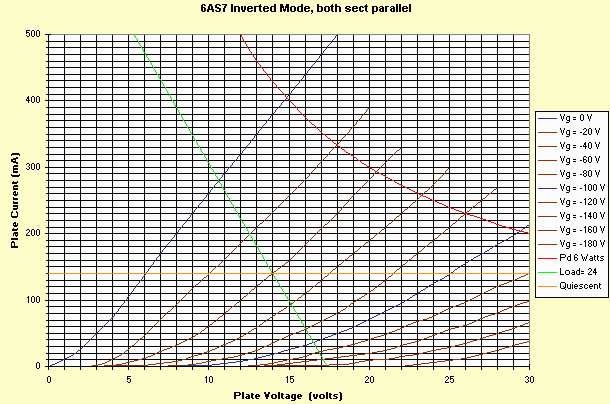In this example, I have "arbitrarily" plotted the maximum power (26 watts in normal operation) as 6 watts (3 watts per section), as the power is dissipated in the grid structure, not the plate. Normally, a tube, even one "spec'd" to handle grid current will have a very limited rating. But this is a spec when the grid is surrounded by a very hot anode. In inverted operation, the plate is negatively biased and does not dissipate power, so the real grid capability is limited by the grid structure. Most low mu power triodes have a fairly "healthy" grid structure, and can thus handle "reasonable" power. Please note that the maximum cathode current (most often listed as maximum plate current) still applies, as this is a function of the cathode structure that emits the electrons.

I have plotted a load line showing 3 tubes in parallel (6 sections) providing over one watt OUTPUT TRANSFORMERLESS AS A SINGLE ENDED AMPLIFIER WITH NO FEEDBACK OR SPECIAL CIRCUITRY. (24 ohm load line).

This you cannot do with the same tubes hooked up in a conventional manner, as the plate resistance is too high to be useful, and the voltage drop across the tube is too high to get any useful current draw!

We have just done an OTL truly single ended amplifier! Note that 80V P-P of drive are required, but since the plate capacitance is lower than the grid capacitance, and there is no appreciable Miller effect, it is actually EASY to drive this amplifier. Also, the power dissipated in each of the grids is about 1 watt; substantially lower than would be required in normal operation. (With 6 to 8 tubes, you can easily get 10 watts, with 12 tubes, over 20 watts of single ended low distortion, well damped output transformerless power). (into 8 ohm load).

For a more adventurous example, let's consider the 5687 small signal triode. This tube has a MU of 15-20, so it will have a very low (.05) MU in inverted mode, but the plate resistance will also be very low. In inverted mode, I have measured the characteristics of some typical 5687's. The results look like this (both sections paralleled).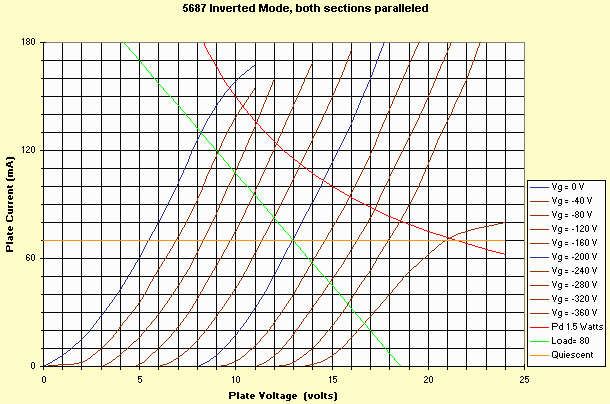Notice 4 things:

1. The linearity is very good; amazingly good in fact.
2. The "plate" resistance is about 45 ohms which is not bad for a cheap tube.
3. The effective mu is about 1/25 (slightly lower than the "estimated" 0.05 for this tube, so the measured plate resistance is actually lower than the "predicted" plate resistance.
4. You can start to see some "fringe" effects at low bias (near zero) and high bias (-360V) conditions. Although beyond the scope of this article, this effect also shows up with EL34 and 6550 operated inverted too. It doesn't show up on the 6AS7, probably due to the lower forward MU.

For this device, I have arbitrarily picked a maximum power per grid of 750 mW (although with the load line I picked, the power dissipated per section is only about 400 mW). I have plotted a load line of 80 ohms (for 10 paralleled tubes this is 8 ohms) - which is still physically small and inexpensive. Notice the very low required voltages required for operation of the output stage. This means we can use the same power supply techniques conventionally used to produce DC heater voltage! The only high voltage required is for the drive, and that is low current! (Although I admit 400 V P-P is a pretty healthy drive signal for a couple watt amp!)

In fact, you can make a practical amplifier from this configuration. The amplifier is described in sections below. If you want a copy of the full schematic it is available at: OTL Test Amplifier Schematic

The amplifier is divided into 3 parts. The first is the input section.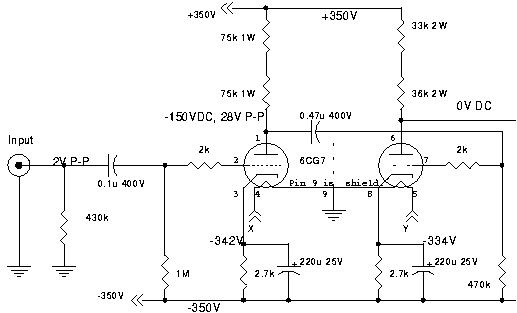This section is merely a drive amplifier for the 5687 output stage. The input stage amplifies the signal from 2 volts to 28 volts p-p. This drives the second stage which amplifies the signal from 28V p-p to 400v p-p. The power supply is +/- 350 volts (700 volt supply), and about 330 volts is placed across the 6CG7, keeping the device within its ratings. The output impedance of this stage is about 7k. The frequency response extends from about 2 Hz to about 200 kHz. The 400V p-p output does include the effects of the 470k load of the output stage.

The output stage is really simple: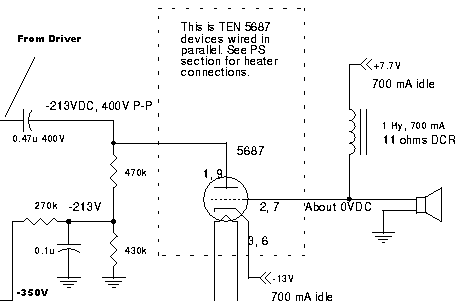The -350 volt supply is voltage divided to set the bias at -200 volts. (-213 to account for the -13 volts on the cathode). The cathode is sent below ground, as 13 volts plate voltage is used quiescent wise, so the DC voltage at the "plate" is zero, and no output coupling capacitor is needed. A 1 Hy is used as a load; this could just as easily be replaced with a 18 ohm resistor if the positive voltage source was changed to +13 volts. The LF response of this stage is about 1 Hz. There are 10 paralleled tubes. For each of the 20 sections, the "input" capacitance ends up being 4.6 pF, for a total of slightly under 100 pF. Driven from a 7k source, the HF response of the output stage is quite good (about 200 kHz). For slew considerations, the "current" is fed from the 70k plate resistor, paralleled with the 470k bias (58.5k). This produces a slew limited response of about 30 kHz, which is quite adequate. The HF actually is limited by the driver, not output. Calculates out to about 150kHz for 3 dB point. Due to the fantastically low gain of the output stage, matching becomes unimportant in the output tubes.

The last portion is the power supply: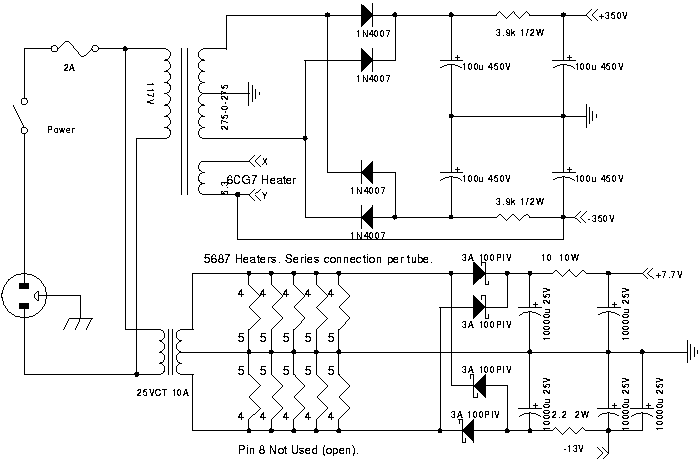The supply is fairly simple. Commonly available transformers are used. Nothing unusual here.

Measured Specifications:

• Frequency Response (1 dB): 3 Hz -50 kHz, 3 Hz - 30 kHz at full power.
• Sensitivity: 1.9V P-P for full power.
• Power Output into 8 ohms: 1.8 watts, before "clipping".
• Distortion: 2.4% at 1.6W, primarily 2nd. (About 1% 3rd, 0.2% 4th.)
• Distortion: 1.1% at 1 watt, again, predominantly 2Nd.
• Output Resistance: about 3.4 ohms.
• Feedback Used: 0 dB.
• Tube lineup: 6CG7 driving 10 paralleled 5687's.

Notice that I used a 1 Hy "choke" in the plate circuit, and a split supply, so that I did not need an output coupling capacitor. You could also replace the choke with a resistor of about 50 ohms, and increase the + supply to supply the 700 mA idle current. These techniques are entirely practical for the low power supply voltages needed in this output stage. Although the required drive is high, the low capacitance of the input (plate) allows relatively high impedance for relatively easy drive.

Two other items to note in the "specs". Although this is a SE OTL circuit with NO FEEDBACK, the damping factor and the frequency response are remarkably good!

How does it sound? As you know, I'm not a particularly big fan of single ended amplifiers, but this one sounds remarkably good. It almost sounds too good for a SE amp. Perhaps it's the relatively high damping factor, and the constant plate resistance (which is definitely more constant than your typical SE amplifier). The response is really great too. Very good bass response from such an anemic amplifier. I ran the test amp from bench supplies, and it's really weird to see plate current kicking to over 1 amp on signal overloads with small tubes! If you're looking for a OTL amp, AND a true SE amp as well, this one might just satisfy you. BTW, if you parallel a few more 5687's, you should be able to reach 5 watts into either 4 or 8 ohms. Caution: This is not a beginners project! There is very high drive voltages present (+/-350 volts), and the circuit looks "odd" for a beginner. This is intended to illustrate inverted tube principles. I have not covered construction techniques, hum source identification and removal, stopper resistors, precautions against shorts etc. Please start with something slightly less exotic first.

Hints on a 20 watt version using 6AS7s

You can get 20 watts SE OTL from 12 paralleled 6AS7s. The only thing that needs changed on the schematic is the power supply and the bias. The bias changes to -120 volts (-152 volts to account for the cathode voltage). The tubes need 32 volts at 170 mA per tube (85 mA per section), so going to a 48V CT transformer is appropriate. This will develop +/- 32 volts. The filaments are arranged across this transformer as 4 series on one side, 4 series on the other side and 4 more series on one of the sides. Drive required is (only) 240V p-p, so you could also lower the 70k plate resistor on the 6CG7 to increase the slew capability. The output choke should probably become a 100 to 500 mH 3 (or so) Amp inductor. Fair Radio has some of these.

I'm not the "inventor" of inverted tube operation. F.E. Terman (Radio Engineers Handbook, etc) described this operation in 1928 in the Proceedings of the IRE. I started experimenting with inverted triodes many years ago. Looking through my old engineering logbooks, I note several tests with a 1626 operated inverted mode done in 1966. That tube did not show any fringe effects at very negative bias levels, but did at near zero bias (forward MU=5 for the 1626). (Well, I DO keep my old logbooks!)

What might be really interesting to try is the Svetlana EL509, as its high gm and high plate current capability implies that only a half dozen of these could deliver pretty high power in OTL. (As this is a beam power tube, tie the screen (g2), the beam formers and the plate (anode) together to form the "grid".)

Steve